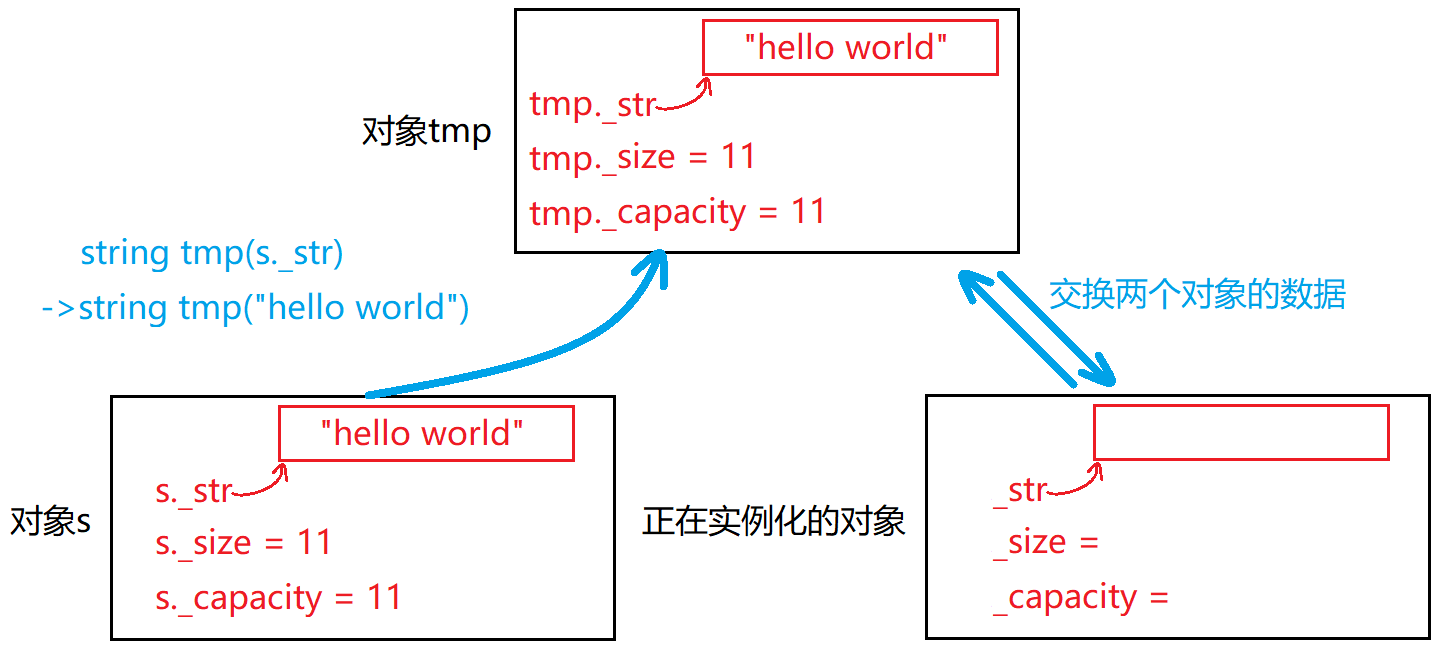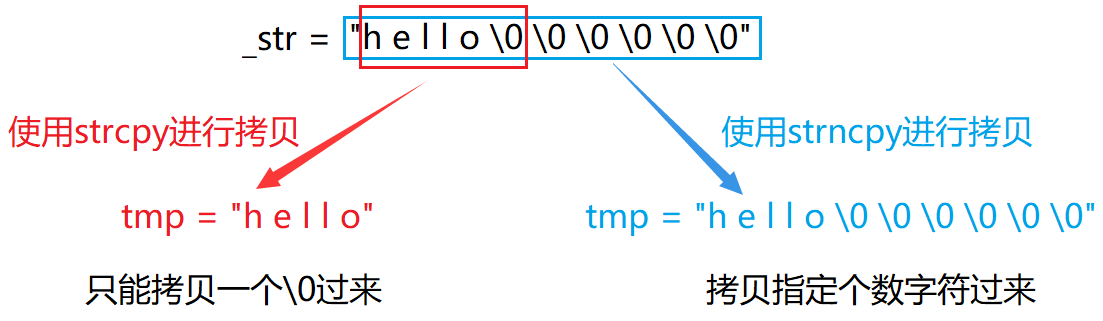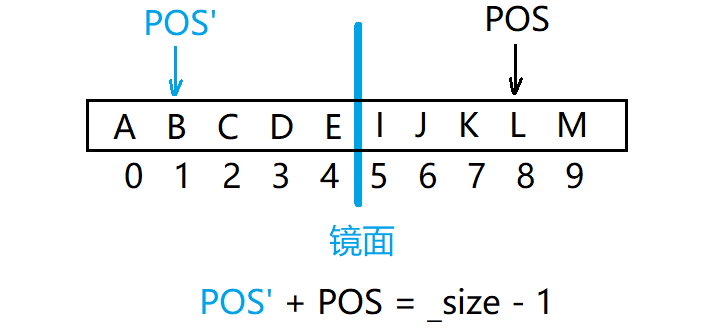# C++STL详解（二）—— string类的模拟实现

### 文章目录

string类各函数接口总览 默认成员函数 构造函数 拷贝构造函数 赋值运算符重载函数 析构函数 迭代器相关函数 begin和end 容量和大小相关函数 size和capacity reserve和resize empty 修改字符串相关函数 push_back append operator+= insert erase clear swap c_str 访问字符串相关函数 operator[ ] find和rfind 关系运算符重载函数 >>和<<运算符的重载以及getline函数 >>运算符的重载 <<运算符的重载 getline

# string类各函数接口总览

``````namespace cl
{
//模拟实现string类
class string
{
public:
typedef char* iterator;
typedef const char* const_iterator;

//默认成员函数
string(const char* str = "");         //构造函数
string(const string& s);              //拷贝构造函数
string& operator=(const string& s);   //赋值运算符重载函数
~string();                            //析构函数

//迭代器相关函数
iterator begin();
iterator end();
const_iterator begin()const;
const_iterator end()const;

//容量和大小相关函数
size_t size();
size_t capacity();
void reserve(size_t n);
void resize(size_t n, char ch = '\0');
bool empty()const;

//修改字符串相关函数
void push_back(char ch);
void append(const char* str);
string& operator+=(char ch);
string& operator+=(const char* str);
string& insert(size_t pos, char ch);
string& insert(size_t pos, const char* str);
string& erase(size_t pos, size_t len);
void clear();
void swap(string& s);
const char* c_str()const;

//访问字符串相关函数
char& operator[](size_t i);
const char& operator[](size_t i)const;
size_t find(char ch, size_t pos = 0)const;
size_t find(const char* str, size_t pos = 0)const;
size_t rfind(char ch, size_t pos = npos)const;
size_t rfind(const char* str, size_t pos = 0)const;

//关系运算符重载函数
bool operator>(const string& s)const;
bool operator>=(const string& s)const;
bool operator<(const string& s)const;
bool operator<=(const string& s)const;
bool operator==(const string& s)const;
bool operator!=(const string& s)const;

private:
char* _str;       //存储字符串
size_t _size;     //记录字符串当前的有效长度
size_t _capacity; //记录字符串当前的容量
static const size_t npos; //静态成员变量（整型最大值）
};
const size_t string::npos = -1;

//<<和>>运算符重载函数
istream& operator>>(istream& in, string& s);
ostream& operator<<(ostream& out, const string& s);
istream& getline(istream& in, string& s);
}
``````

# 默认成员函数

## 构造函数

``````//构造函数
string(const char* str = "")
{
_size = strlen(str); //初始时，字符串大小设置为字符串长度
_capacity = _size; //初始时，字符串容量设置为字符串长度
_str = new char[_capacity + 1]; //为存储字符串开辟空间（多开一个用于存放'\0'）
strcpy(_str, str); //将C字符串拷贝到已开好的空间
}
``````

## 拷贝构造函数``````//传统写法
string(const string& s)
:_str(new char[strlen(s._str) + 1]) //_str申请一块刚好可以容纳s._str的空间
, _size(0)
, _capacity(0)
{
strcpy(_str, s._str);    //将s._str拷贝一份到_str
_size = s._size;         //_size赋值
_capacity = s._capacity; //_capacity赋值
}
````````````//现代写法
string(const string& s)
:_str(nullptr)
, _size(0)
, _capacity(0)
{
string tmp(s._str); //调用构造函数，构造出一个C字符串为s._str的对象
swap(tmp); //交换这两个对象
}
``````

## 赋值运算符重载函数

``````//传统写法
string& operator=(const string& s)
{
if (this != &s) //防止自己给自己赋值
{
delete[] _str; //将原来_str指向的空间释放
_str = new char[strlen(s._str) + 1]; //重新申请一块刚好可以容纳s._str的空间
strcpy(_str, s._str);    //将s._str拷贝一份到_str
_size = s._size;         //_size赋值
_capacity = s._capacity; //_capacity赋值
}
return *this; //返回左值（支持连续赋值）
}
``````

``````//现代写法1
string& operator=(string s) //编译器接收右值的时候自动调用拷贝构造函数
{
swap(s); //交换这两个对象
return *this; //返回左值（支持连续赋值）
}
``````

``````//现代写法2
string& operator=(const string& s)
{
if (this != &s) //防止自己给自己赋值
{
string tmp(s); //用s拷贝构造出对象tmp
swap(tmp); //交换这两个对象
}
return *this; //返回左值（支持连续赋值）
}
``````

## 析构函数

string类的析构函数需要我们进行编写，因为每个string对象中的成员_str都指向堆区的一块空间，当对象销毁时堆区对应的空间并不会自动销毁，为了避免内存泄漏，我们需要使用delete手动释放堆区的空间。

``````//析构函数
~string()
{
delete[] _str;  //释放_str指向的空间
_str = nullptr; //及时置空，防止非法访问
_size = 0;      //大小置0
_capacity = 0;  //容量置0
}
``````

# 迭代器相关函数

string类中的迭代器实际上就是字符指针，只是给字符指针起了一个别名叫iterator而已。

``````typedef char* iterator;
typedef const char* const_iterator;
``````

## begin和end

string类中的begin和end函数的实现简单的可怕，begin函数的作用就是返回字符串中第一个字符的地址：

``````iterator begin()
{
return _str; //返回字符串中第一个字符的地址
}
const_iterator begin()const
{
return _str; //返回字符串中第一个字符的const地址
}
``````

end函数的作用就是返回字符串中最后一个字符的后一个字符的地址（即’\0’的地址）：

``````iterator end()
{
return _str + _size; //返回字符串中最后一个字符的后一个字符的地址
}
const_iterator end()const
{
return _str + _size; //返回字符串中最后一个字符的后一个字符的const地址
}
``````

``````string s("hello world!!!");
string::iterator it = s.begin();
while (it != s.end())
{
cout << *it << " ";
it++;
}
cout << endl;
``````

``````string s("hello world!!!");
//编译器将其替换为迭代器形式
for (auto e : s)
{
cout << e << " ";
}
cout << endl;
``````

# 容量和大小相关函数

## size和capacity

size函数用于获取字符串当前的有效长度（不包括’\0’）。

``````//大小
size_t size()const
{
return _size; //返回字符串当前的有效长度
}
``````

capacity函数用于获取字符串当前的容量。

``````//容量
size_t capacity()const
{
return _capacity; //返回字符串当前的容量
}
``````

## reserve和resize

reserve和resize这两个函数的执行规则一定要区分清楚。
reserve规则：
1、当n大于对象当前的capacity时，将capacity扩大到n或大于n。
2、当n小于对象当前的capacity时，什么也不做。

``````//改变容量，大小不变
void reserve(size_t n)
{
if (n > _capacity) //当n大于对象当前容量时才需执行操作
{
char* tmp = new char[n + 1]; //多开一个空间用于存放'\0'
strncpy(tmp, _str, _size + 1); //将对象原本的C字符串拷贝过来（包括'\0'）
delete[] _str; //释放对象原本的空间
_str = tmp; //将新开辟的空间交给_str
_capacity = n; //容量跟着改变
}
}
``````resize规则：
1、当n大于当前的size时，将size扩大到n，扩大的字符为ch，若ch未给出，则默认为’\0’。
2、当n小于当前的size时，将size缩小到n。

``````//改变大小
void resize(size_t n, char ch = '\0')
{
if (n <= _size) //n小于当前size
{
_size = n; //将size调整为n
_str[_size] = '\0'; //在size个字符后放上'\0'
}
else //n大于当前的size
{
if (n > _capacity) //判断是否需要扩容
{
reserve(n); //扩容
}
for (size_t i = _size; i < n; i++) //将size扩大到n，扩大的字符为ch
{
_str[i] = ch;
}
_size = n; //size更新
_str[_size] = '\0'; //字符串后面放上'\0'
}
}
``````

## empty

empty是string的判空函数，我们可以调用strcmp函数来实现，strcmp函数是用于比较两个字符串大小的函数，当两个字符串相等时返回0。

``````//判空
bool empty()
{
return strcmp(_str, "") == 0;
}
``````

# 修改字符串相关函数

## push_back

push_back函数的作用就是在当前字符串的后面尾插上一个字符，尾插之前首先需要判断是否需要增容，若需要，则调用reserve函数进行增容，然后再尾插字符，注意尾插完字符后需要在该字符的后方设置上’\0’，否则打印字符串的时候会出现非法访问，因为尾插的字符后方不一定就是’\0’。

``````//尾插字符
void push_back(char ch)
{
if (_size == _capacity) //判断是否需要增容
{
reserve(_capacity == 0 ? 4 : _capacity * 2); //将容量扩大为原来的两倍
}
_str[_size] = ch; //将字符尾插到字符串
_str[_size + 1] = '\0'; //字符串后面放上'\0'
_size++; //字符串的大小加一
}
``````

``````//尾插字符
void push_back(char ch)
{
insert(_size, ch); //在字符串末尾插入字符ch
}
``````

## append

append函数的作用是在当前字符串的后面尾插一个字符串，尾插前需要判断当前字符串的空间能否容纳下尾插后的字符串，若不能，则需要先进行增容，然后再将待尾插的字符串尾插到对象的后方，因为待尾插的字符串后方自身带有’\0’，所以我们无需再在后方设置’\0’。

``````//尾插字符串
void append(const char* str)
{
size_t len = _size + strlen(str); //尾插str后字符串的大小（不包括'\0'）
if (len > _capacity) //判断是否需要增容
{
reserve(len); //增容
}
strcpy(_str + _size, str); //将str尾插到字符串后面
_size = len; //字符串大小改变
}
``````

``````//尾插字符串
void append(const char* str)
{
insert(_size, str); //在字符串末尾插入字符串str
}
``````

## operator+=

+=运算符的重载是为了实现字符串与字符、字符串与字符串之间能够直接使用+=运算符进行尾插。
+=运算符实现字符串与字符之间的尾插直接调用push_back函数即可。

``````//+=运算符重载
string& operator+=(char ch)
{
push_back(ch); //尾插字符串
return *this; //返回左值（支持连续+=）
}
``````

+=运算符实现字符串与字符串之间的尾插直接调用append函数即可。

``````//+=运算符重载
string& operator+=(const char* str)
{
append(str); //尾插字符串
return *this; //返回左值（支持连续+=）
}
``````

## insert

insert函数的作用是在字符串的任意位置插入字符或是字符串。
insert函数用于插入字符时，首先需要判断pos的合法性，若不合法则无法进行操作，紧接着还需判断当前对象能否容纳插入字符后的字符串，若不能则还需调用reserve函数进行扩容。插入字符的过程也是比较简单的，先将pos位置及其后面的字符统一向后挪动一位，给待插入的字符留出位置，然后将字符插入字符串即可。``````//在pos位置插入字符
string& insert(size_t pos, char ch)
{
assert(pos <= _size); //检测下标的合法性
if (_size == _capacity) //判断是否需要增容
{
reserve(_capacity == 0 ? 4 : _capacity * 2); //将容量扩大为原来的两倍
}
char* end = _str + _size;
//将pos位置及其之后的字符向后挪动一位
while (end >= _str + pos)
{
*(end + 1) = *(end);
end--;
}
_str[pos] = ch; //pos位置放上指定字符
_size++; //size更新
return *this;
}
``````

insert函数用于插入字符串时，首先也是判断pos的合法性，若不合法则无法进行操作，再判断当前对象能否容纳插入该字符串后的字符串，若不能则还需调用reserve函数进行扩容。插入字符串时，先将pos位置及其后面的字符统一向后挪动len位（len为待插入字符串的长度），给待插入的字符串留出位置，然后将其插入字符串即可。``````//在pos位置插入字符串
string& insert(size_t pos, const char* str)
{
assert(pos <= _size); //检测下标的合法性
size_t len = strlen(str); //计算需要插入的字符串的长度（不含'\0'）
if (len + _size > _capacity) //判断是否需要增容
{
reserve(len + _size); //增容
}
char* end = _str + _size;
//将pos位置及其之后的字符向后挪动len位
while (end >= _str + pos)
{
*(end + len) = *(end);
end--;
}
strncpy(_str + pos, str, len); //pos位置开始放上指定字符串
_size += len; //size更新
return *this;
}
``````

## erase

erase函数的作用是删除字符串任意位置开始的n个字符。删除字符前也需要判断pos的合法性，进行删除操作的时候分两种情况：
1、pos位置及其之后的有效字符都需要被删除。2、pos位置及其之后的有效字符只需删除一部分。``````//删除pos位置开始的len个字符
string& erase(size_t pos, size_t len = npos)
{
assert(pos < _size); //检测下标的合法性
size_t n = _size - pos; //pos位置及其后面的有效字符总数
if (len >= n) //说明pos位置及其后面的字符都被删除
{
_size = pos; //size更新
_str[_size] = '\0'; //字符串后面放上'\0'
}
else //说明pos位置及其后方的有效字符需要保留一部分
{
strcpy(_str + pos, _str + pos + len); //用需要保留的有效字符覆盖需要删除的有效字符
_size -= len; //size更新
}
return *this;
}
``````

## clear

clear函数用于将对象中存储的字符串置空，实现时直接将对象的_size置空，然后在字符串后面放上’\0’即可。

``````//清空字符串
void clear()
{
_size = 0; //size置空
_str[_size] = '\0'; //字符串后面放上'\0'
}
``````

## swap

swap函数用于交换两个对象的数据，直接调用库里的swap模板函数将对象的各个成员变量进行交换即可。但我们若是想在这里调用库里的swap模板函数，需要在swap函数之前加上“::”（作用域限定符），告诉编译器优先在全局范围寻找swap函数，否则编译器编译时会认为你调用的是正在实现的swap函数（就近原则）。

``````//交换两个对象的数据
void swap(string& s)
{
//调用库里的swap
::swap(_str, s._str); //交换两个对象的C字符串
::swap(_size, s._size); //交换两个对象的大小
::swap(_capacity, s._capacity); //交换两个对象的容量
}
``````

## c_str

c_str函数用于获取对象C类型的字符串，实现时直接返回对象的成员变量_str即可。

``````//返回C类型的字符串
const char* c_str()const
{
return _str;
}
``````

# 访问字符串相关函数

## operator[ ]

[ ]运算符的重载是为了让string对象能像C字符串一样，通过[ ] +下标的方式获取字符串对应位置的字符。

``````//[]运算符重载（可读可写）
char& operator[](size_t i)
{
assert(i < _size); //检测下标的合法性
return _str[i]; //返回对应字符
}
``````

``````//[]运算符重载（只读）
const char& operator[](size_t i)const
{
assert(i < _size); //检测下标的合法性
return _str[i]; //返回对应字符
}
``````

## find和rfind

find函数和rfind函数都是用于在字符串中查找一个字符或是字符串，find函数和rfind函数分别用于正向查找和反向查找，即从字符串开头开始向后查找和从字符串末尾开始向前查找。
find函数：
1、正向查找第一个匹配的字符。

``````//正向查找第一个匹配的字符
size_t find(char ch, size_t pos = 0)
{
assert(pos < _size); //检测下标的合法性
for (size_t i = pos; i < _size; i++) //从pos位置开始向后寻找目标字符
{
if (_str[i] == ch)
{
return i; //找到目标字符，返回其下标
}
}
return npos; //没有找到目标字符，返回npos
}
``````

2、正向查找第一个匹配的字符串。

``````//正向查找第一个匹配的字符串
size_t find(const char* str, size_t pos = 0)
{
assert(pos < _size); //检测下标的合法性
const char* ret = strstr(_str + pos, str); //调用strstr进行查找
if (ret) //ret不为空指针，说明找到了
{
return ret - _str; //返回字符串第一个字符的下标
}
else //没有找到
{
return npos; //返回npos
}
}
``````

rfind函数：1、反向查找第一个匹配的字符。

``````//反向查找第一个匹配的字符
size_t rfind(char ch, size_t pos = npos)
{
string tmp(*this); //拷贝构造对象tmp
reverse(tmp.begin(), tmp.end()); //调用reverse逆置对象tmp的C字符串
if (pos >= _size) //所给pos大于字符串有效长度
{
pos = _size - 1; //重新设置pos为字符串最后一个字符的下标
}
pos = _size - 1 - pos; //将pos改为镜像对称后的位置
size_t ret = tmp.find(ch, pos); //复用find函数
if (ret != npos)
return _size - 1 - ret; //找到了，返回ret镜像对称后的位置
else
return npos; //没找到，返回npos
}
``````

2、反向查找第一个匹配的字符串。

``````//反向查找第一个匹配的字符串
size_t rfind(const char* str, size_t pos = npos)
{
string tmp(*this); //拷贝构造对象tmp
reverse(tmp.begin(), tmp.end()); //调用reverse逆置对象tmp的C字符串
size_t len = strlen(str); //待查找的字符串的长度
char* arr = new char[len + 1]; //开辟arr字符串（用于拷贝str字符串）
strcpy(arr, str); //拷贝str给arr
size_t left = 0, right = len - 1; //设置左右指针
//逆置字符串arr
while (left < right)
{
::swap(arr[left], arr[right]);
left++;
right--;
}
if (pos >= _size) //所给pos大于字符串有效长度
{
pos = _size - 1; //重新设置pos为字符串最后一个字符的下标
}
pos = _size - 1 - pos; //将pos改为镜像对称后的位置
size_t ret = tmp.find(arr, pos); //复用find函数
delete[] arr; //销毁arr指向的空间，避免内存泄漏
if (ret != npos)
return _size - ret - len; //找到了，返回ret镜像对称后再调整的位置
else
return npos; //没找到，返回npos
}
``````

# 关系运算符重载函数

``````//>运算符重载
bool operator>(const string& s)const
{
return strcmp(_str, s._str) > 0;
}
//==运算符重载
bool operator==(const string& s)const
{
return strcmp(_str, s._str) == 0;
}
``````

``````//>=运算符重载
bool operator>=(const string& s)const
{
return (*this > s) || (*this == s);
}
//<运算符重载
bool operator<(const string& s)const
{
return !(*this >= s);
}
//<=运算符重载
bool operator<=(const string& s)const
{
return !(*this > s);
}
//!=运算符重载
bool operator!=(const string& s)const
{
return !(*this == s);
}
``````

# >>和<<运算符的重载以及getline函数

## >>运算符的重载

``````//>>运算符的重载
istream& operator>>(istream& in, string& s)
{
s.clear(); //清空字符串
char ch = in.get(); //读取一个字符
while (ch != ' '&&ch != '\n') //当读取到的字符不是空格或'\n'的时候继续读取
{
s += ch; //将读取到的字符尾插到字符串后面
ch = in.get(); //继续读取字符
}
return in; //支持连续输入
}
``````

## <<运算符的重载

``````//<<运算符的重载
ostream& operator<<(ostream& out, const string& s)
{
//使用范围for遍历字符串并输出
for (auto e : s)
{
cout << e;
}
return out; //支持连续输出
}
``````

## getline

getline函数用于读取一行含有空格的字符串。实现时于>>运算符的重载基本相同，只是当读取到’\n’的时候才停止读取字符。

``````//读取一行含有空格的字符串
istream& getline(istream& in, string& s)
{
s.clear(); //清空字符串
char ch = in.get(); //读取一个字符
while (ch != '\n') //当读取到的字符不是'\n'的时候继续读取
{
s += ch; //将读取到的字符尾插到字符串后面
ch = in.get(); //继续读取字符
}
return in;
}
``````